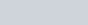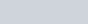The sum of n terms of an A.P. is 3n2 + 5n, then 164 is its
Question:

The sum of $n$ terms of an A.P. is $3 n^{2}+5 n$, then 164 is its

(a) 24th term

(b) 27th term

(c) 26th term

(d) 25th term

Solution:

Here, the sum of first n terms is given by the expression,We need to find which term of the A.P. is 164.

Let us take 164 as the nth term.

So we know that the nthterm of an A.P. is given by,So,

$164=S_{n}-S_{n-1}$

$164=3 n^{2}+5 n-\left[3(n-1)^{2}+5(n-1)\right]$

Using the property,

$(a-b)^{2}=a^{2}+b^{2}-2 a b$

We get,

$164=3 n^{2}+5 n-\left[3\left(n^{2}+1-2 n\right)+5(n-1)\right]$

$164=3 n^{2}+5 n-\left[3 n^{2}+3-6 n+5 n-5\right]$

$164=3 n^{2}+5 n-\left(3 n^{2}-n-2\right)$

$164=3 n^{2}+5 n-3 n^{2}+n+2$

$164=6 n+2$

Further solving for n, we get

$6 n=164-2$

$n=\frac{162}{6}$

$n=27$

Therefore, 164 is the $27^{\text {th }}$ term of the given A.P.

Hence the correct option is (b).